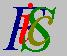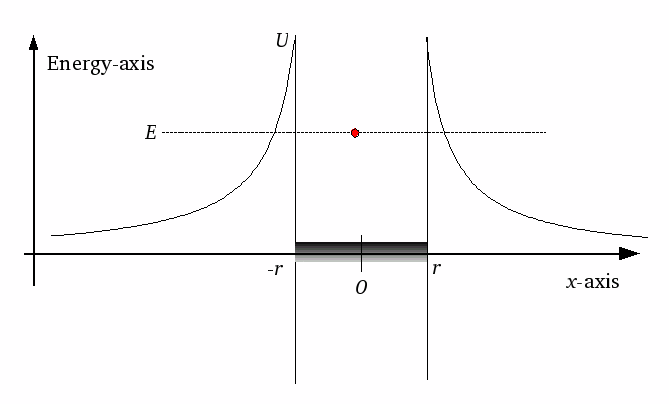Mauro Murzi's pages on Philosophy of Science - Quantum mechanicsTransmission coefficient Commutative operators.# [4. Applications]

The alpha decay is a type of radioactive disintegration whereby a parent nucleus, with charge Ze (e is the electron charge), breaks up into a decay nucleus, called the daughter, with charge (Z-2)e, and a helium nucleus, called alpha particle, with charge 2e.

The alpha decay can be explained in terms of the tunnel effect. The figure is a drastically simplified illustration of the situation.An alpha particle (red dot), with energy E, is inside a nucleus (gray region of x-axis), with radius r. Outside the nucleus (where | x | > r) the potential U is the Coulomb potential: U = 2e² (Z - 2) / | x |. When the alpha particle is outside the nucleus, its measured energy E is less than the maximum value of U = 2e² (Z - 2) / | r |. Thus the alpha particle is inside a potential well and, according to classical mechanics, its energy is insufficient to escape.

The explanation of alpha radioactivity is possible in terms of the tunnel effect, according to which there is a not null probability that the alpha particle can move through the potential barrier, escaping from the nucleus.

The main difficulty in explaining alpha radioactivity arise from the very large variability of the half life of the emitting substances. From the empirical evidence results that the half life depends on the energy of the emitted alpha particle. This energy varies between 4 MeV and 10 MeV (thus a variation by a factor 2.5) while the half life varies between 1010 years (=1018 seconds) and 0.1 μsec (=10-7 seconds), with a variation by a factor 1025. How so a large variation can be explained, when the energy varies by a small factor?

The transmission coefficient, that is the probability that the alpha particle escapes from the nucleus, is correlated with the half life of the emitting nucleus. The theory of the tunnel effect enables us to calculate the transmission coefficient; using some drastic simplifications, the following relation, which connects the half life τ and the energy E of the alpha particle, can be obtained:

(4.2)    Log(τ) = (148 / √E) - 53.5

where τ is measured in seconds and E in MeV. Calculating the equation (4.2) for the values E = 4 MeV and E = 10 MeV we obtain:

for E = 4 MeV : Log(τ) = (148 /√4 ) - 53.5 = 20.5
for E = 10 MeV : Log(τ) = (148 /√10 ) - 53.5 = -6.7

The logarithm of the half life shows a variation of about 27; thus the half life shows a variation by a factor 1027. Hence a small variation in the energy of the alpha particle can explain the very large amount of variability of the half life of the emitting substances.Transmission coefficient Commutative operators.